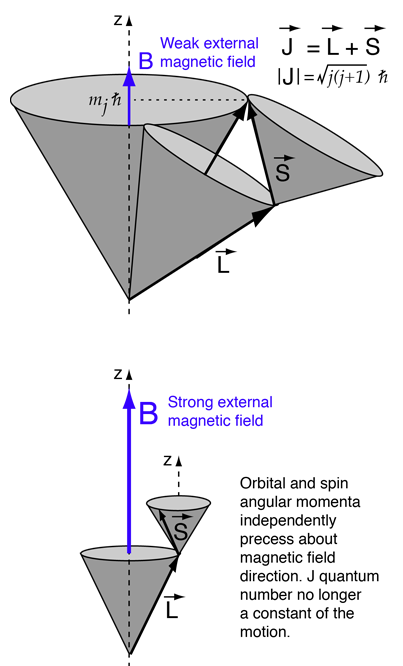# Paschen-Back Effect

In the presense of an external magnetic field, the energy levels of atoms are split. This splitting is described well by the Zeeman effect if the splitting is small compared to the energy difference between the unperturbed levels, i.e., for sufficiently weak magnetic fields. This can be visualized with the help of a vector model of total angular momentum. If the magnetic field is large enough, it disrupts the coupling between the orbital and spin angular momenta, resulting in a different pattern of splitting. This effect is called the Paschen-Back effect.In the weak field case the vector model at left implies that the coupling of the orbital angular momentum L to the spin angular momentum S is stronger than their coupling to the external field. In this case where spin-orbit coupling is dominant, they can be visualized as combining to form a total angular momentum J which then precesses about the magnetic field direction. In the strong-field case, S and L couple more strongly to the external magnetic field than to each other, and can be visualized as independently precessing about the external field direction.

For reference, the sodium Zeeman effect is reproduced below to show the nature of the magnetic interaction for weak external magnetic fields.The following is a model of the changes in the pattern if the magnetic field were strong enough to decouple L and S. The resulting spectrum would be a triplet with the center line twice the intensity of the outer lines.To create this pattern, the projections of L and S in the z-direction have been treated independently and the ms multiplied by the spin g-factor. The energy shift is expressed as a multiple of the Bohr magneton mB. The selection rules explain why the transitions shown are allowed and others not.

Sodium was used as the basis of the model for convenience, but the fields required to create Paschen-Back conditions for sodium are unrealistically high. Lithium, on the other hand, has a spin-orbit splitting of only 0.00004 eV compared to 0.0021 eV for sodium. Such small energy values are sometimes expressed in "wavenumbers", or 1/l in cm-1. In these units the lithium separation is about 0.3 cm-1and the sodium separation is about 17 cm-1 .The Paschen-Back conditions are met in some lithium spectra observed on the Sun, so this effect does have astronomical significance.

Index

Hydrogen concepts

 HyperPhysics***** Quantum Physics R Nave
Go Back